Courses

# Gas Power Cycles - MCQ Test 2

## 30 Questions MCQ Test Mock Test Series - Mechanical Engineering (ME) for GATE 2020 | Gas Power Cycles - MCQ Test 2

Description
This mock test of Gas Power Cycles - MCQ Test 2 for Mechanical Engineering helps you for every Mechanical Engineering entrance exam. This contains 30 Multiple Choice Questions for Mechanical Engineering Gas Power Cycles - MCQ Test 2 (mcq) to study with solutions a complete question bank. The solved questions answers in this Gas Power Cycles - MCQ Test 2 quiz give you a good mix of easy questions and tough questions. Mechanical Engineering students definitely take this Gas Power Cycles - MCQ Test 2 exercise for a better result in the exam. You can find other Gas Power Cycles - MCQ Test 2 extra questions, long questions & short questions for Mechanical Engineering on EduRev as well by searching above.
QUESTION: 1

### The cycle which consists of two reversible isotherms and two reversible isochores is called as

Solution:
*Multiple options can be correct
QUESTION: 2

Solution:

Ans. A,D

QUESTION: 3

### Which one of the following cycles has the highest thermal efficiency for given maximum and minimum cycle temperatures?

Solution:

Ans. (d) Don’t confuse with Diesel cycle. As stirling cycle’s efficiency is equal to Carnot cycle.

QUESTION: 4

The data given in the table refers to an engine based on Carnot cycle, whereQ1 = Heat received (kJ/min), Q2 = Heat rejected (kJ/s), W = Work output (kW)
S. No.                     Q1                         Q2                           W
1.                          1500                 16.80                    8.20
2.                          1600                 17.92                    8.75
3.                          1700                  19.03                   9.30
4.                          1800                   20.15                  9.85
If heat received by the engine is 2000 kJ/minute the work output will be,nearly,

Solution:

Ans. (d)

QUESTION: 5

Which one of the following changes/sets of changes in the source and sink temperatures (T1 and T2 respectively) of a reversible engine will result in the maximum improvement in efficiency?

Solution:

Ans. (c) For maximum improvement in efficiency source temperature should be raised and sink temperature lowered.

QUESTION: 6

What does the reversed ideal Stirling cycle consist of?

Solution:

Ans. (b)

QUESTION: 7

Which one of the following parameters is significant to ascertain chemicalequilibrium of a system?

Solution:

Ans. (c)

QUESTION: 8

For maximum specific output of a constant volume cycle (Otto cycle)

Solution:

Ans. (d)

QUESTION: 9

For the same maximum pressure and heat input, the most efficient cycle is

Solution:

Ans. (b)

QUESTION: 10

Assertion (A): Power generated by a four stroke engine working on Otto cycle is higher than the power generated by a two stroke engine for the same swept volume, speed,temperature and pressure conditions.

Reason (R): In a four stroke engine one cycle is completed in two revolutions.

Solution:

Ans. (d)

QUESTION: 11

Match List I with II and select the correct answer using the code given below the
Lists:

List I                                                     List II
(Prime Mover)                                   (Air Standard Cycle)
A. High Speed diesel engine             1. Atkinson Cycle
B. IC engine having expansion          2. Dual combustion limited pressure ratio greater than compression Praagtieo  of cycle
C. Pulse jet engine                             3. Erickson Cycle
D. Gas turbine with multistage           4. Lenoir cycle compression and multistage expansion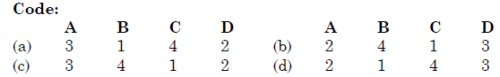Solution:

Ans. (d)

QUESTION: 12

Match List-I with List-II and select the correct answer using the codes given below the lists: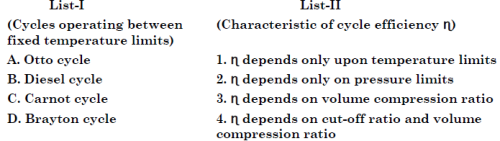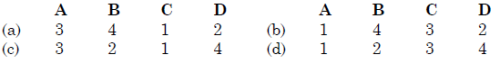Solution:

Ans. (a)

QUESTION: 13

Assertion (A): In practice, the efficiency of diesel engines is higher than that of petrolengines.
Reason (R): For the same compression ratio, the efficiency of diesel cycle is higherthan that of Otto cycle.

Solution:

Ans. (c)

QUESTION: 14

Match List I (Cycles) with List II (Processes) and select the correct answer using the codes given below the Lists: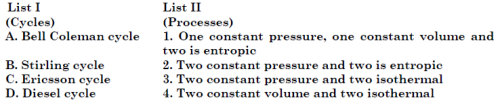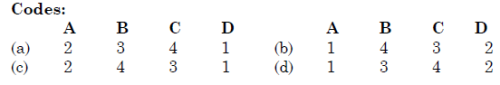Solution:

Ans. (c)

QUESTION: 15

A gas turbine cycle with infinitely large number of stages during compressionand expansion approaches

Solution:

Ans. (c)

QUESTION: 16

In the case of a Diesel cycle, increasing the cut-off ratio will increase

Solution:
QUESTION: 17

Assertion (A): For a given compression ratio, the thermal efficiency of the Diesel cycle will be higher than that of the Otto cycle.
Reason(R): In the Diesel cycle, work is also delivered during heat addition.

Solution:

Ans. (d) For same compression ratio Otto cycle has maximum efficiency.

QUESTION: 18

The air standard efficiency of diesel cycle will be less than that of Otto cyclein the case of

Solution:

Ans. (a)

QUESTION: 19

Match List I with List II and select the correct answer using the codes given below the lists: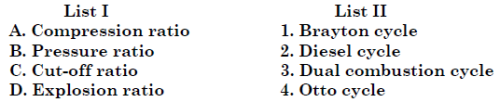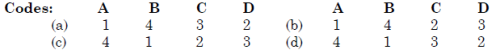Solution:

Ans. (c)

QUESTION: 20

For the same compression ratio and the same heat input, the correctsequence of the increasing order of the thermal efficiencies of the givencycles is

Solution:

Ans. (b)

QUESTION: 21

Otto cycle efficiency is higher than Diesel cycle efficiency for the same compression ratio and heat input because, in Otto cycle

Solution:

Ans. (d) For same heat input and same compression ratio, in case of Otto cycle, efficiency in higher because the heat rejection is lower.

QUESTION: 22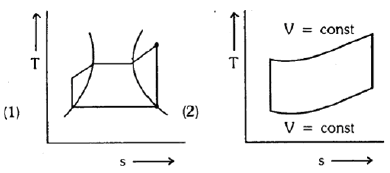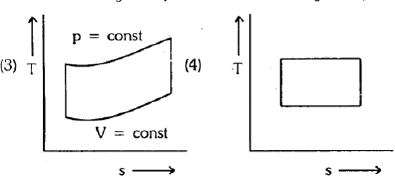The correct sequence of the given four cycles on T-s plane in Figure (1), (2),(3), (4) is

Solution:

Ans. (b)

QUESTION: 23

Which one of the following hypothetical heat engine cycle represents maximum efficiency?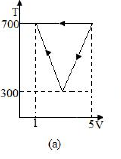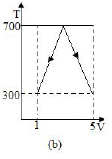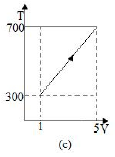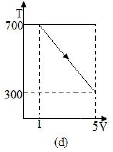Solution:

Ans. (b)

Work done by all cases is same =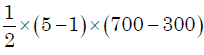= 800 units

Heat input for (a) and (c) is same = 700 x (5 - 1) =  280 units

Heat input for(b)is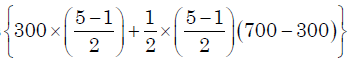= 1000 units

Heat input for(d)is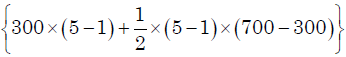= 2000 units

∴ maximum when minimum heat input is case (b)

QUESTION: 24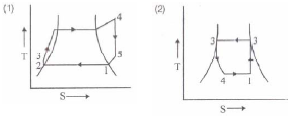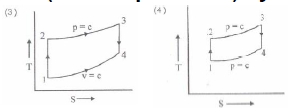The correct sequence of the cycles given in the above T-S diagrams is

Solution:

Ans. (b)

QUESTION: 25

Stirling and Ericsson cycles are

Solution:

Ans. (c)

QUESTION: 26

In an air standard Otto cycle, r is the volume compression ratio and y is an adiabatic index (Cp/ Cv), the air standard p v efficiency is given by

Solution:

Ans. (a)

QUESTION: 27

Which one of the following p-T diagrams illustrates the Otto cycle of an ideal gas?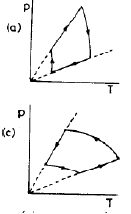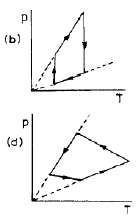Solution:

Ans. (a)

QUESTION: 28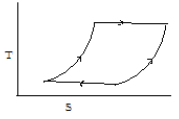Thermodynamic cycle shown above on the temperature – entropy diagrampertains to which one of the following?

Solution:

Ans. (a) 1-2 and 3-4 are isothermal process
2-3 and 4-1 may be isobaric or isochoric process
So this cycle may be Starling cycle of Ericsson cycle but steepness of the curve 2-3 and 1-4 is very high. Therefore we may say it is Starling cycle.

QUESTION: 29

A cyclic heat engine does 50 kJ of work per cycle. If the efficiency of the heat engine is 75%, the heat rejected per cycle is

Solution:

Ans. (a)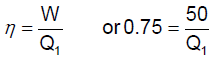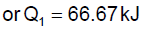and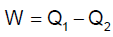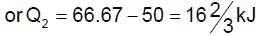QUESTION: 30

A solar energy based heat engine which receives 80 kJ of heat at 100 deg Cand rejects 70 kJ of heat to the ambient at 30 deg C is to be designed. The thermal efficiency of the heat engine is

Solution:

Ans. (c)